### Home > CAAC > Chapter 14 > Lesson 14.3.1.6 > Problem3-86

3-86.
1. Place the triangle below on a set of x and y axes so that B is located at the origin. Homework Help ✎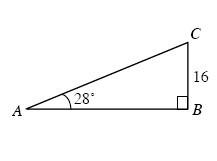1. Find the coordinates of A and C.

2. Find the area and perimeter of triangle ABC.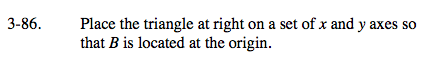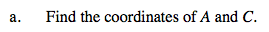Use the trigonometric ratios to find the other side lengths.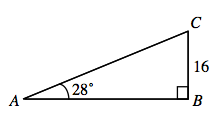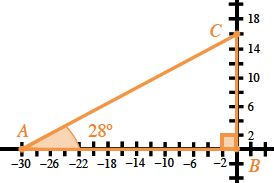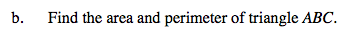Find the missing side lengths using the trigonometric ratios and then use those to find the area and perimeter.